# What Is a Current Limiting Resistor?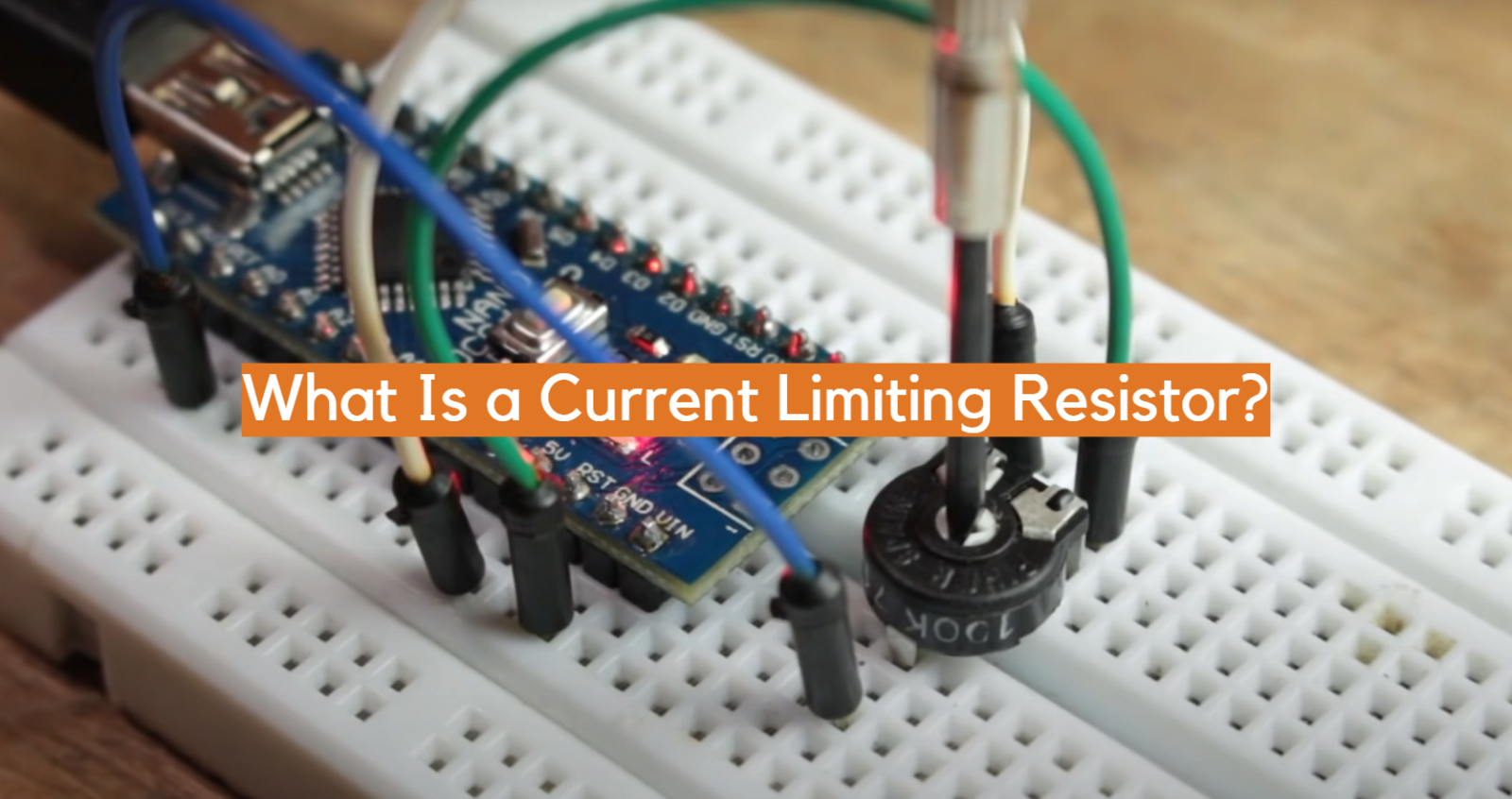In the vast realm of electronics, electrical components play a crucial role in enabling the functionality and safety of various devices. Among these components, the current limiting resistor holds a significant position, serving as a fundamental building block for countless electronic circuits. Acting as a guardian of current flow, a current-limiting resistor acts as a vital safeguard against excessive current, preventing damage to components and ensuring the overall stability and longevity of electronic systems.

In this article, we delve into the intricacies of current limiting resistors, exploring their purpose, working principle, and practical applications. We examine the factors to consider when selecting a resistor, such as resistance value, power rating, and tolerance. Furthermore, we explore various circuit configurations that employ current-limiting resistors and discuss their importance in protecting LEDs, integrated circuits (ICs), and other electronic devices.

Ultimately, by unraveling the mysteries surrounding current limiting resistors, readers will gain a comprehensive understanding of this critical component, empowering them to design robust circuits and ensure the safety and longevity of their electronic systems.

## A Current Limiting Resistor Definition

At its core, a current-limiting resistor is a passive electronic component designed to regulate the flow of electric current through a circuit. It accomplishes this by providing resistance to the flow of electrons, impeding the current, and keeping it within safe operational limits. This simple yet essential function makes the current limiting resistor a staple in numerous applications, ranging from consumer electronics to industrial machinery .Understanding the principle of Ohm’s Law is crucial to comprehend the role of a current-limiting resistor. According to Ohm’s Law, the current passing through a conductor is directly proportional to the voltage across it and inversely proportional to the resistance in the circuit. By manipulating the resistance value, a current-limiting resistor restricts the current passing through a circuit, acting as a control mechanism.

The significance of current limiting resistors becomes particularly evident when interfacing electronic components with varying current requirements. In such scenarios, the resistor serves as a bridge, matching the current output of a power source with the input capability of a receiving device. By providing the necessary resistance, the current limiting resistor ensures the circuit operates within its safe limits, shielding sensitive components from potential damage caused by excessive current flow.

## Why Current Limiting Resistors Are Necessary for LED Light Circuits?

Current limiting resistors are necessary for LED light circuits in order to prevent the LED from becoming damaged due to excessive current. The voltage drop across an LED remains relatively constant over a wide range of operating currents, so a small increase in voltage can lead to a large increase in current. By using a current-limiting resistor, the amount of current flowing through the LED can be controlled and kept within safe limits .

Without a current-limiting resistor, the LED could be damaged or even destroyed due to overheating.

Simply put, using a current limiting resistor ensures the stable and reliable operation of the LED circuit.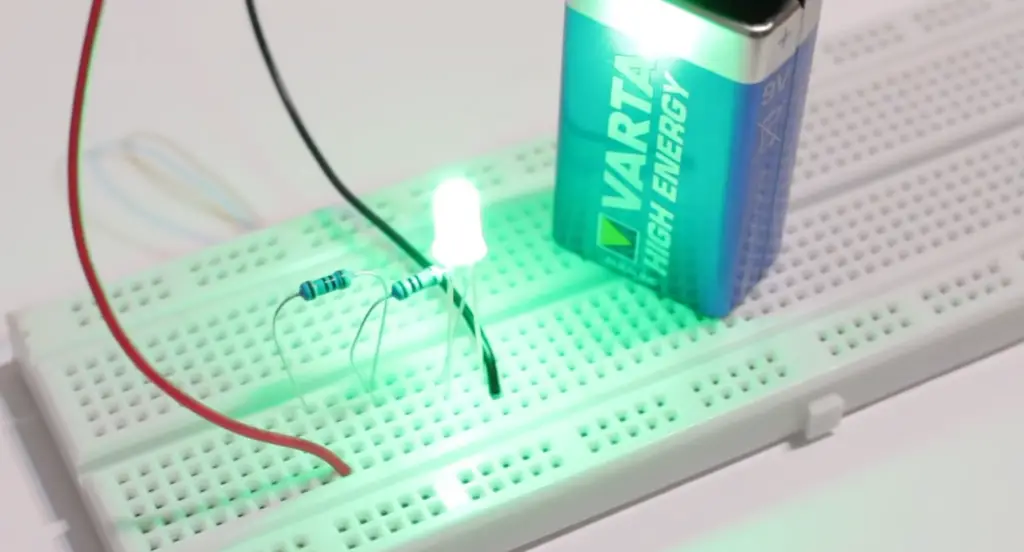## How Does Current Limiting Resistor Work?

A current-limiting resistor works by impeding the flow of electric current through a circuit, thereby regulating and restricting the amount of current that can pass through. It achieves this by providing resistance, which is measured in ohms (Ω), to the flow of electrons.

According to Ohm’s Law, the current (I) passing through a circuit is directly proportional to the voltage (V) across it and inversely proportional to the resistance (R) in the circuit. The equation can be represented as I = V/R. By manipulating the resistance value, a current-limiting resistor can control the amount of current flowing through the circuit.

When a current limiting resistor is added to a circuit, it creates a voltage drop across itself. This voltage drop is proportional to the amount of current flowing through the resistor and the resistance value. By using resistors with specific resistance values, it is possible to achieve the desired current limitation.

## The Role of Current Limiting Resistor

The concept of current limiting is particularly important in electronic circuits that involve components with specific current requirements. For example, light-emitting diodes (LEDs) have a maximum current rating, and exceeding this rating can lead to premature failure. By connecting an appropriate current-limiting resistor in series with an LED, the resistor restricts the current flowing through the LED, preventing it from being damaged by excessive current.

In addition to protecting components, current limiting resistors also play a crucial role in maintaining circuit stability and preventing overloading. By limiting the current, resistors prevent excessive power dissipation and reduce the risk of overheating. They act as safeguards against short circuits and other electrical faults, preventing damage to the power source and the connected components.

When selecting a current-limiting resistor, several factors must be considered, including the desired current limitation, power rating, tolerance, and temperature coefficient. By choosing the right resistor for a specific application, engineers can ensure that the circuit operates safely and efficiently.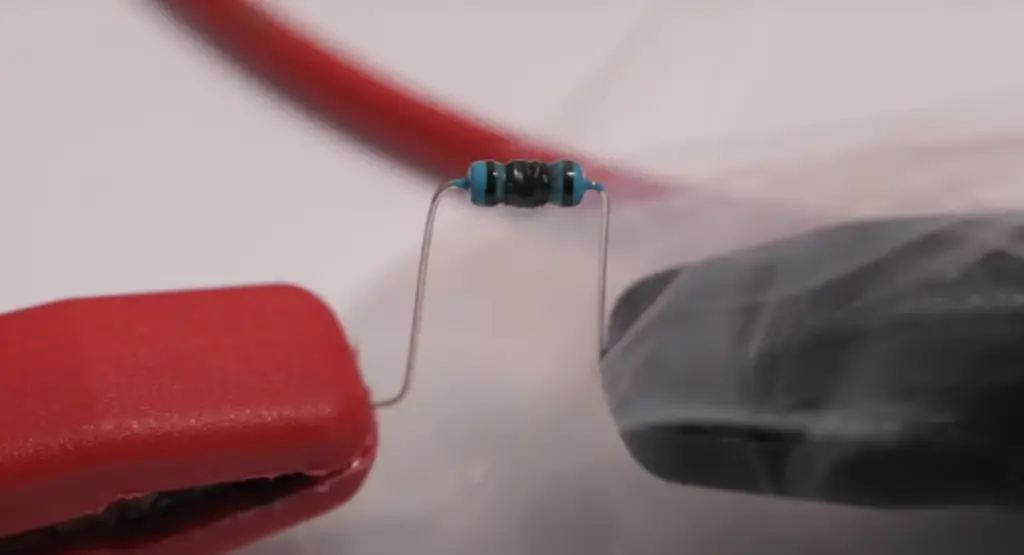## Specific Examples of the Function of Current Limiting Resistor

A current-limiting resistor is an essential component in many electronic circuits, and there are numerous specific examples of its function.

One example is in LED light circuits where the resistor limits the current through the LED, preventing it from overheating or getting damaged by excess current. Another example is in power supply circuits, where the resistor helps limit the current through a power transistor, protecting it from failure due to overheating.

In audio circuits, current limiting resistors are used to isolate the audio signal from DC voltages and to prevent amplifier circuits from being overloaded.

In addition, current limiting resistors are commonly used in motor control circuits to prevent stall conditions and other dangerous high-current situations. In some types of power management circuits, such as load switches, MOSFET transistors are used with external resistors to set the current limit threshold, which helps protect the load and the circuitry that is generating or transmitting the current.

The function of current limiting resistors is to ensure the safe and stable operation of electronic circuits by controlling the amount of current flowing through key components. By limiting the amount of current, these resistors help prevent damage to sensitive electronic components and preserve the reliability and longevity of the circuit.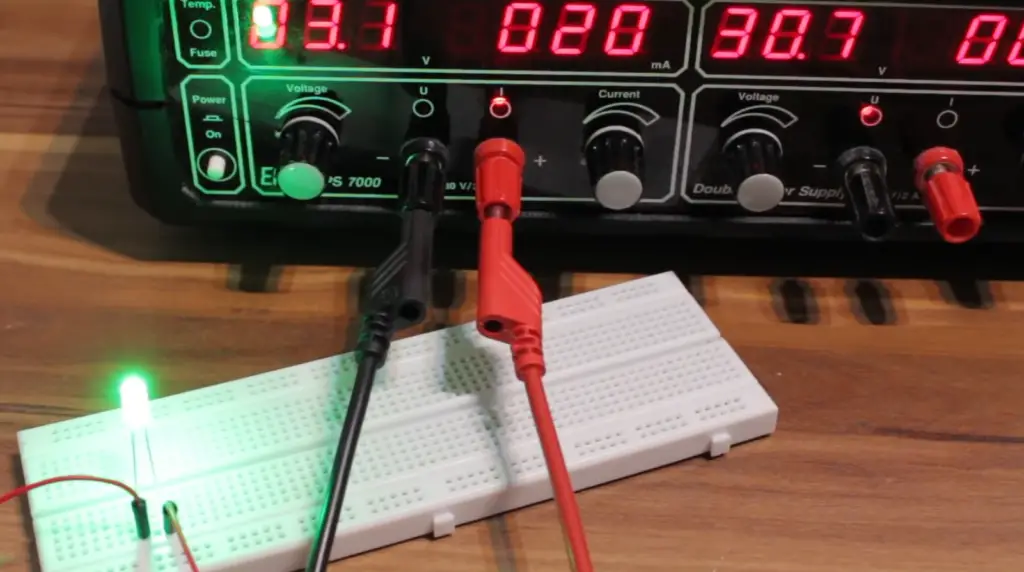## Cause of Burning Current Limiting Resistor

• Incorrect Resistance Value: Using a current-limiting resistor with an incorrect resistance value can result in inadequate current restriction. If the resistance is too low, it may not provide enough impedance to limit the current effectively. Conversely, if the resistance is too high, it may excessively restrict the current, causing voltage drops and potentially leading to overheating;
• Overvoltage: Applying a voltage higher than the resistor’s maximum rating can cause excessive current flow and lead to resistor burning. Voltage spikes, power surges, or incorrect power supply connections can result in overvoltage conditions that surpass the resistor’s capabilities;
• Excessive Load: Connecting a load that demands more current than the current limiting resistor can handle may overload the resistor. This can occur when multiple components are connected in parallel or when the load itself has a low impedance, effectively bypassing the resistor’s limiting function;
• Manufacturing Defects: In rare cases, manufacturing defects in the resistor can lead to abnormal behavior, including increased resistance or inadequate heat dissipation. These defects can cause the resistor to burn under normal operating conditions;
• Overheating: Inadequate heat dissipation can cause the resistor’s temperature to rise beyond its maximum tolerance, leading to burning. Factors such as high ambient temperature, insufficient airflow, improper mounting, or inadequate heat sinking can contribute to overheating;
• Circuit Design Errors: Mistakes in circuit design, such as incorrect component placement, improper wiring, or incorrect calculations, can result in unintended high currents flowing through the resistor. These errors can lead to resistor burnout if the current exceeds the resistor’s power rating ;

To prevent the burning of current-limiting resistors, it is essential to select resistors with appropriate resistance values, power ratings, and voltage tolerances. Careful circuit design, adherence to specifications, and thorough testing can help identify and rectify potential issues before they cause damage. Additionally, considering factors like heat dissipation, load requirements, and voltage stability can contribute to maintaining the resistor within its safe operating limits.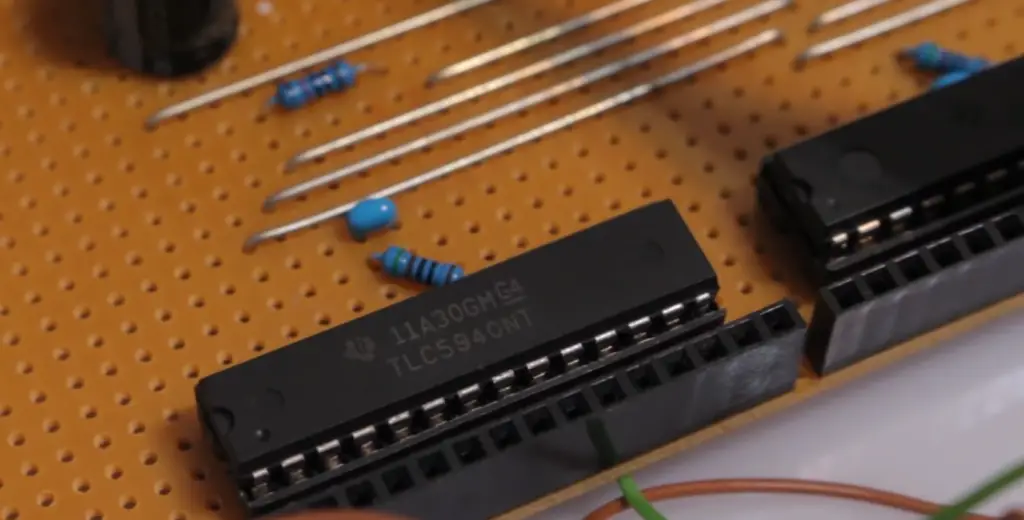## How to Choose A Current Limiting Resistor: Tips

Choosing the right current limiting resistor for a specific circuit can be challenging, but there are some tips that can help.

Firstly, it is important to know the operating voltage and current of the device or component that the resistor is being used with. This information can be obtained from the device’s datasheet or manual.

Once you know the operating voltage and current, you can use Ohm’s law to calculate the resistance needed for the current limiting resistor. The formula for resistance is R = V/I, where R is resistance, V is voltage, and I is current.

When choosing a resistor, it is also important to consider the power rating of the resistor. The power rating tells you how much power the resistor can safely dissipate without getting too hot and possibly failing. To calculate the power rating for a current limiting resistor, use the formula P = I^2 * R, where P is power, I is current, and R is resistance .

Lastly, it’s also essential to consider the tolerance of the resistor. Resistor tolerance is the maximum allowable deviation in resistance from the stated value. A lower tolerance value means a resistor’s actual resistance is more likely to be close to the stated value.

## Calculating Current Limiting Resistor Values for LED Circuits:

### Single LEDs

To calculate the current limiting resistor value for a single LED, you need to consider the LED’s forward voltage (Vf) and the desired current (If). The formula to determine the resistor value is:

R = (Vsource – Vf) / If

Where R is the resistor value in ohms, Vsource is the voltage source (supply voltage), Vf is the forward voltage of the LED, and If is the desired current.

For example, if you have a 5V supply voltage, an LED with a forward voltage of 2V, and you want a current of 20mA (0.02A) flowing through the LED, the resistor value would be:

R = (5V – 2V) / 0.02A = 150 ohms

Choose the nearest standard resistor value, such as 150 ohms or the next available higher value, to obtain the desired current limitation.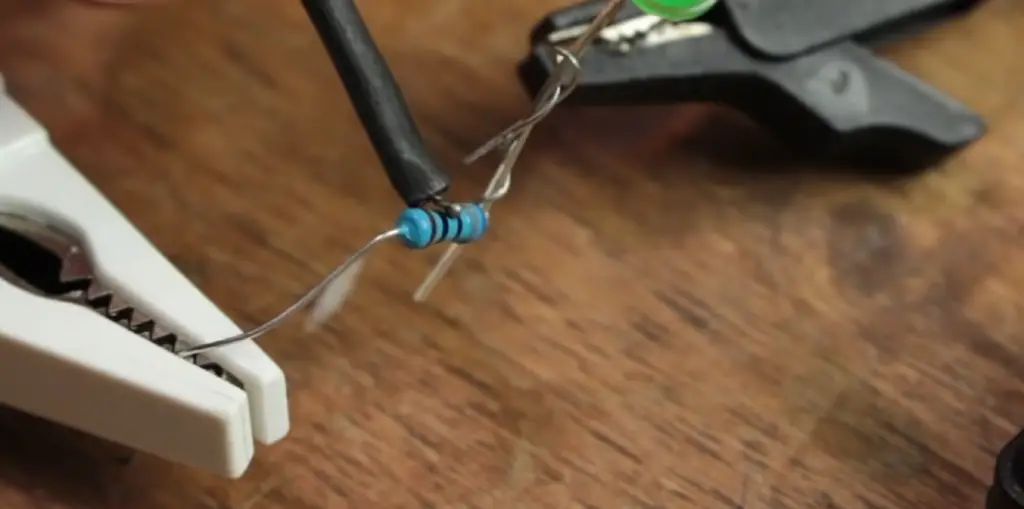### LEDs in Series

When connecting multiple LEDs in series, the total forward voltage across the LEDs adds up. To calculate the current limiting resistor value, follow these steps:

1. Determine the total forward voltage (Vtotal) across the series LEDs;
2. Calculate the resistor value using the formula mentioned in the single LED calculation, considering the supply voltage and Vtotal;

For example, if you have three LEDs in series, each with a forward voltage of 2V, and you want a current of 20mA flowing through them, and a 5V supply voltage, the total forward voltage would be 6V (3 x 2V).

Using the same formula as in the single LED calculation, the resistor value would be:

R = (5V – 6V) / 0.02A = -50 ohms

Since a negative resistor value is not possible, you would need to adjust the supply voltage or the number of LEDs to obtain a positive resistor value .

### LEDs in Parallel

When connecting LEDs in parallel, each LED will have its own current-limiting resistor. This ensures that the current is divided equally among the LEDs. Calculate the resistor value for each LED independently using the single LED calculation method.

### Current Limiting Resistor: Controlling Brightness

Apart from protecting the LED, a current-limiting resistor can also be used to control the LED’s brightness. By adjusting the resistor value, you can vary the current flowing through the LED and consequently control its brightness level. Higher resistor values result in lower currents and dimmer LEDs, while lower resistor values lead to higher currents and brighter LEDs.

When calculating resistor values for brightness control, consider the desired brightness level, the LED’s forward voltage, and the supply voltage. Experimentation may be required to achieve the desired brightness, as it depends on the specific LED and personal preference.

Remember to use standard resistor values that are commercially available. If the calculated value is not available, choose the next higher value to ensure the current limitation is not compromised.

By accurately calculating current limiting resistor values for LED circuits, you can ensure proper operation, protect the LEDs from excessive current, and control the brightness to meet your requirements. These calculations form the foundation for designing reliable and efficient LED circuits.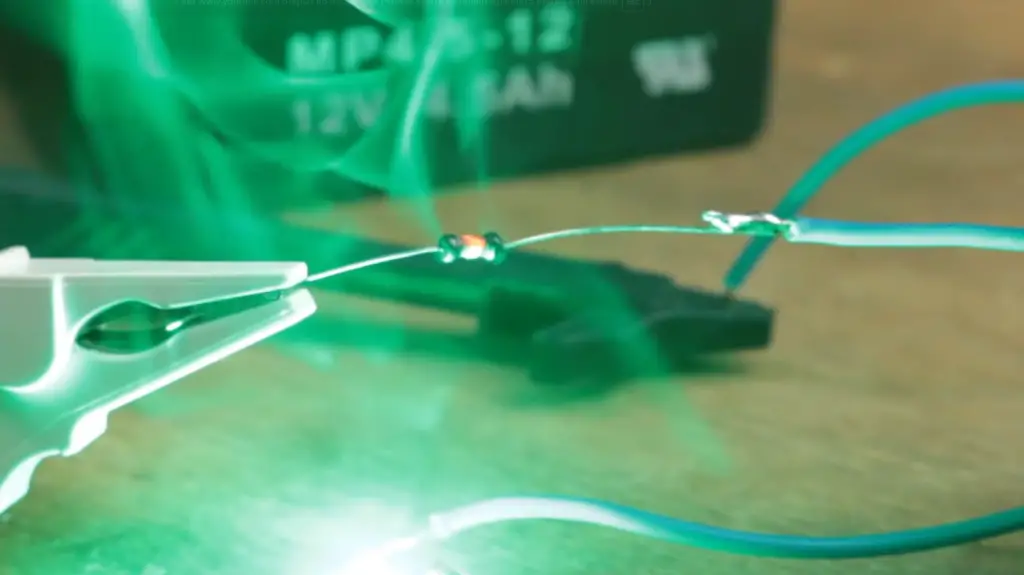## How to Choose the LED Current Limiting Resistor:

1. Determine the LED specifications: Start by gathering information about the LED, including its forward voltage (Vf) and maximum forward current (If). This information is typically provided in the LED datasheet or product specifications;
2. Determine the power supply voltage: Identify the voltage of the power supply that will be driving the LED circuit. This voltage should be within the acceptable range for the LED and the desired brightness level;
3. Calculate the desired LED current: Decide on the desired current (If) that should flow through the LED for optimal performance and brightness. This value should be within the LED’s maximum forward current rating;
4. Calculate the resistor value: Use Ohm’s Law (V = I * R) to calculate the resistor value (R) based on the LED’s forward voltage and the desired current. Rearrange the formula as R = (Vsupply – Vf) / If, where Vsupply is the power supply voltage;
5. Choose the nearest standard resistor value: Based on the calculated resistor value, select the nearest standard resistor value available commercially. It is recommended to choose a resistor with a slightly higher resistance value to ensure that the current remains within the desired range and to provide a safety margin;
6. Check power rating: Ensure that the chosen resistor has an appropriate power rating. The power dissipated by the resistor can be calculated using the formula P = I^2 * R, where P is the power in watts, I is the current in amperes, and R is the resistance in ohms. Select a resistor with a power rating higher than the calculated power to avoid overheating and potential failure;
7. Verify tolerance: Consider the resistor’s tolerance, which indicates the allowable deviation from the specified resistance value. Typically, resistors have tolerances of 5% or 1%. Ensure that the chosen resistor’s tolerance does not significantly affect the desired current or brightness level;
8. Heat dissipation: Assess the heat dissipation requirements for the resistor. If the LED circuit is expected to operate at higher currents or for extended periods, consider using a resistor with a higher power rating or implementing heat sinks or other cooling measures to prevent overheating;
9. Practical considerations: Finally, consider practical aspects such as the physical size and availability of the resistor, especially if you are planning to assemble the circuit on a PCB or breadboard ;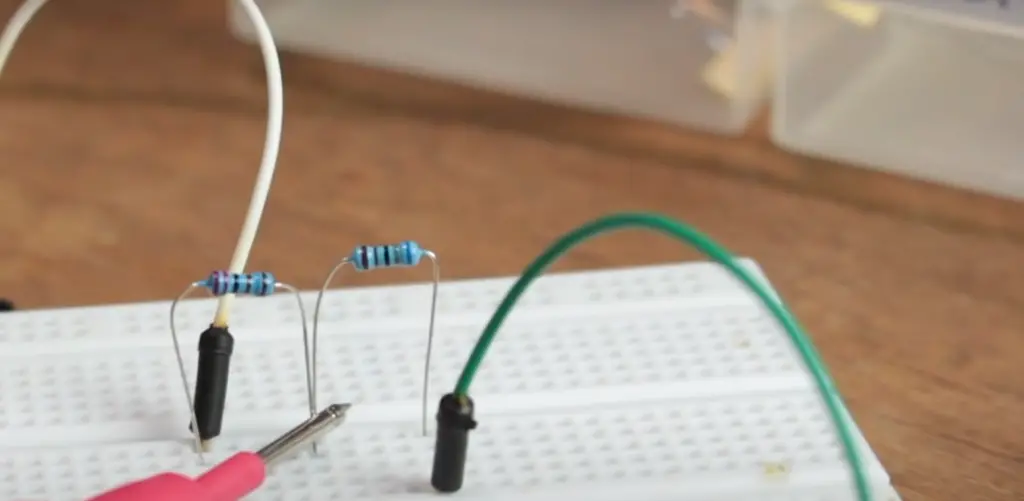## How to Choose the Zener Diode Current Limiting Resistor?

To choose the Zener diode current limiting resistor, you’ll need to take into account the maximum current that will flow through the Zener diode and the voltage drop across it. The goal is to choose a resistor value that will limit the current to a safe level while still allowing the Zener diode to work properly.

The formula for calculating the resistor value is R = (Vs – Vz) / Iz, where Vs is the supply voltage, Vz is the Zener voltage, and Iz is the Zener current. Once you know the resistor value, you can choose the next highest standard value that is available .

However, in real-world applications, there may be additional factors to consider, such as the power dissipation of the resistor and the temperature coefficient of the Zener diode. In these cases, it may be necessary to use a larger resistor or a Zener diode with a different voltage rating to ensure proper operation and reliability.

Consulting the datasheets for the Zener diode and the resistor can provide more information and guidance on selecting the appropriate components for a given circuit.

## FAQ

### 1. Do you need a current limiting resistor?

In many electronic circuits, it is necessary to include a current-limiting resistor. The need for a current-limiting resistor depends on the specific components and their current requirements.

For example, light-emitting diodes (LEDs) typically require a current-limiting resistor to prevent excessive current flow, which can lead to their failure.

Similarly, when interfacing components with different current handling capabilities, such as a power source and a load, a current limiting resistor may be necessary to match the current output of the source to the input requirements of the load.

### 2. What is the purpose of a current limiter?

The purpose of a current limiter is to regulate and restrict the amount of electric current flowing through a circuit.

It protects components from damage caused by excessive current, prevents overloading and overheating, and ensures the circuit operates within its safe operational limits. Current limiters, such as current limiting resistors, are essential for maintaining the stability, longevity, and reliability of electronic systems.

### 3. What is a MOSFET current-limiting resistor?

A MOSFET current-limiting resistor refers to a current-limiting resistor used in conjunction with a MOSFET (Metal-Oxide-Semiconductor Field-Effect Transistor). MOSFETs are commonly used as electronic switches or amplifiers in various circuits. A current-limiting resistor connected in series with a MOSFET limits the current flowing through it, preventing excessive current that could damage the MOSFET or the connected components .

### 4. What is the main motive for using current limiting resistors?

The main motive for using current limiting resistors is to protect electronic components and ensure safe and reliable operation. Current limiting resistors control and restrict the amount of current flowing through a circuit, preventing excessive current that could damage components such as LEDs, integrated circuits, or other sensitive devices. They also help prevent overloading, overheating, and voltage drops, ensuring the circuit operates within its safe operational limits.

### 5. Do you need a resistor for every LED?

Yes, it is generally necessary to use a resistor for each LED in a circuit. LEDs have specific voltage and current requirements, and exceeding these values can cause them to fail. To ensure the LED operates within its safe current range, a current limiting resistor is used in series with the LED. The resistance value of the resistor is calculated based on the LED’s forward voltage and desired current, ensuring that the LED receives the appropriate amount of current and preventing excessive current flow.

### 6. Why do LEDs need current limiting resistors?

LEDs need current-limiting resistors to regulate the current flowing through them. LEDs are designed to operate within a specific current range, and exceeding this range can result in overheating and premature failure. The forward voltage of an LED remains relatively constant, and by using a current-limiting resistor, the resistor drops the excess voltage and restricts the current to the desired level. This ensures the LED receives the appropriate current and protects it from damage due to excessive current flow.

### 7. What happens when you don’t connect a limiting resistor in the LED circuit?

When a limiting resistor is not connected in an LED circuit, the LED may receive an excessive amount of current, potentially causing it to burn out. Without the resistor, the LED’s forward voltage is not adequately controlled, leading to a higher current flow than the LED can handle. This can result in overheating and permanent damage to the LED, shortening its lifespan or causing immediate failure. Therefore, it is crucial to include a current limiting resistor to ensure the LED operates within its safe current range and to protect it from damage.

### 8. What resistor do I need for a 5V LED?

To determine what resistor you need for a 5V LED, you’ll need to know the forward voltage and current rating for the LED. Once you have this information, you can use Ohm’s Law to calculate the appropriate resistance.

The formula is R = (5V – Vf) / If, where R is the resistance value (in ohms), Vf is the forward voltage of the LED, and If is the desired current for the LED (in amperes). A typical value for the current of an LED is around 20mA.

### 9. Can 2 LEDs share a resistor?

It is generally not recommended to share a resistor between 2 LEDs because the LEDs may have slightly different forward voltage and current ratings, and this can cause one LED to be brighter than the other. Additionally, if one LED fails, the other LED will be without a current limiting resistor and may fail as well.

### 10. What are the 4 types of resistors?

There are 4 main types of resistors: carbon composition, film, wire wound, and surface mount. Each type has different characteristics and features that make it suitable for different applications.

### 11. What is the difference between resistance and resistor?

Resistance is a physical property of a material or device that resists the flow of electric current. It is measured in ohms (Ω). A resistor is an electronic component designed to provide a specific resistance value in a circuit and is typically made up of a length of wire or other material with a specific resistance value.

### 13. Will a 9V battery power a 12V LED?

No, a 9V battery will not power a 12V LED. The LED requires a voltage that is greater than its forward voltage drop in order to operate, and the voltage of a 9V battery is not high enough to power a 12V LED .

### 14. What is the current limit of LEDs?

The current limit of LEDs depends on the specific LED and its datasheet specifications. Typically, the maximum continuous forward current for a standard LED is around 20mA.

### 15. Do LEDs always need a resistor?

Yes, LEDs typically require a current-limiting resistor to prevent them from being damaged by excessive current. However, in some specialized circuits, such as constant current LED drivers, the need for an external resistor may be eliminated.

### 16. Why should you use a 330-Ohm resistor for LEDs?

A 330-ohm resistor is a commonly used value for current-limiting resistors in LED circuits because it provides adequate current limiting for most standard LEDs. This value is commonly used with 5V power sources and LEDs that have a forward voltage of around 2V. Using a resistor of this value helps to ensure that the LED operates within safe current limits and doesn’t get damaged from excessive current.

### 17. What happens if my resistor is too high?

If the resistor value in a circuit is too high, it will limit the current to levels that may be too low to properly power or operate the device or component. This can cause the device to not function properly or not function at all. Additionally, having a resistor that’s too high will also cause the device or component to receive less power than needed, which can lead to reduced performance or failure over time.